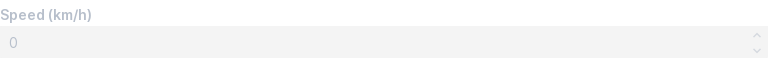# Spinbox¶

Use spinboxes to capture numeric inputs. A spinbox lets users incrementally adjust a value in small steps.

## Basic¶

Call `box()` with `mode='number'` to show a box with increment/decrement buttons. (also called a spinbox).

``````speed = view(box('Speed (km/h)', mode='number'))
``````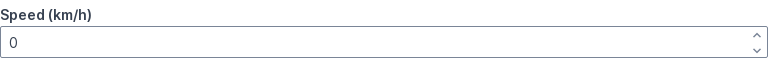## Set initial value¶

Set `value=` to a numeric value to prefill the box with the value.

The mode setting `mode='number'` is implied, and can be elided.

``````speed = view(box('Speed (km/h)', value=42))
``````

In other words, calling `box()` with a numeric `value` has the same effect as setting `mode='number'`, and is the preferred usage.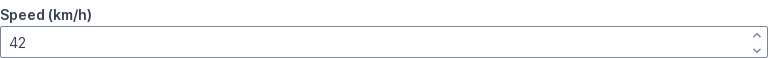## Set min value¶

Set `min=` to specify a minimum value.

``````speed = view(box('Speed (km/h)', min=10))
``````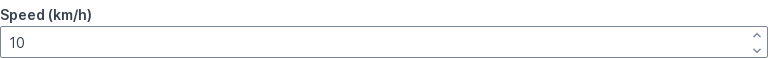## Set max value¶

Set `max=` to specify a maximum value.

``````speed = view(box('Speed (km/h)', max=100))
``````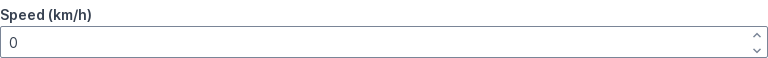## Set step¶

Set `step=` to specify how much to increment or decrement by.

The default step is `1`.

``````speed = view(box('Speed (km/h)', step=5))
``````## Set precision¶

Set `precision=` to specify how many decimal places the value should be rounded to.

The default is calculated based on the precision of step:

• if step = 1, precision = 0
• if step = 0.42, precision = 2
• if step = 0.0042, precision = 4
``````speed = view(box('Speed (m/s)', value=0.6, min=-2, max=2, step=0.2, precision=2))
``````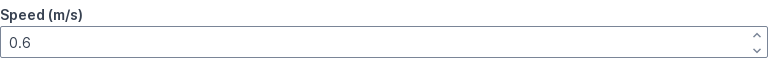## Combine min, max, step, precision¶

`min=`, `max=`, `step=` and `precision=` can be combined.

``````speed = view(box('Speed (km/h)', min=10, max=100, step=5))
``````## Set range¶

Set `range=` to a `(min, max)` tuple to restrict numeric inputs between two values.

This is a shorthand notation for setting `min=` and `max=` individually.

``````speed = view(box('Speed (km/h)', range=(10, 100)))
``````## Set range with step¶

Set `range=` to a `(min, max, step)` tuple to increment/decrement by steps other than `1`.

This is a shorthand notation for setting `min=`, `max=` and `step` individually.

``````speed = view(box('Speed (km/h)', range=(10, 100, 5)))
``````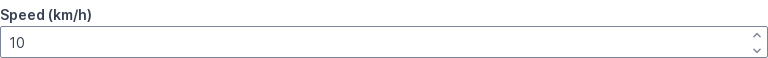## Set range with precision¶

Setting `range=` to a `(min, max, step, precision)` tuple is a shorthand notation for setting `min=`, `max=`, `step` and `precision` individually.

``````speed = view(box('Speed (m/s)', value=0.6, range=(-2, 2, 0.2, 2)))
``````## Use zero-crossing ranges¶

Ranges can cross zero.

``````speed = view(box('Speed (m/s)', value=-3, range=(-5, 5)))
``````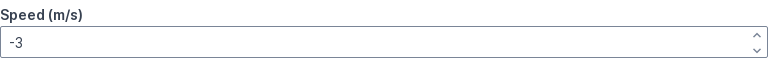## Use fractional steps¶

Steps can be fractional.

``````speed = view(box('Speed (m/s)', value=0.6, range=(-2, 2, 0.2)))
``````## Handle changes immediately¶

Add `live` to `mode` to handle changes immediately.

``````speed = 42  # Starting value
while True:
speed = view(
box('Speed (km/h)', mode='live', value=speed),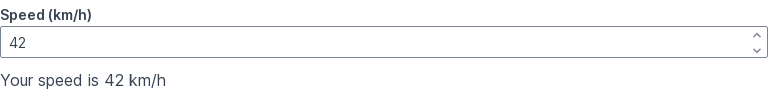Set `disabled=True` to disable.
``````view(box('Speed (km/h)', mode='number', disabled=True))